| Next || Previous || Top |

## Example 9--Kinetic Oxidation of Dissolved Ferrous Iron With Oxygen

Kinetic rate expressions can be defined in a completely general way in PHREEQC by using Basic statements in the RATES data block. The rate expressions can be used in batch-reaction and transport calculations with the KINETICS data block. For transport calculations (ADVECTION or TRANSPORT), kinetic reactions can be defined cell by cell by the number range following the KINETICS keyword (KINETICS m-n ). The rate expressions are integrated with an embedded (up to) 5th-order Runge-Kutta-Fehlberg algorithm, or with a stiff, variable-order, variable-step multistep solver (Cohen and Hindmarsh, 1996). Equilibrium is calculated before a kinetic calculation is initiated and again when a kinetic reaction increment is added. Equilibrium includes solution species equilibrium; exchange-, equilibrium-phase-, solid-solution-, and surface-assemblage equilibrium; and gas-phase equilibrium. A check is performed to ensure that the difference between estimates of the integrated rate over a time interval is smaller than a user-defined tolerance. If the tolerance is not satisfied, then the integration over the time interval is automatically restarted with a smaller time interval.

Kinetic reactions between solids and the aqueous phase can be calculated without modification of the database. PHREEQC also can calculate kinetic reactions among aqueous species that are normally assumed to be in equilibrium, but this requires that the database be redefined with separate SOLUTION_MASTER_SPECIES for the aqueous species that react kinetically. Example 9 illustrates the procedure for decoupling two valence states of an element (iron) and shows how PHREEQC can be used to calculate the kinetic oxidation of Fe +2 to Fe +3 in water.

The rate of oxidation of Fe +2 by O 2 in water is given by (Singer and Stumm, 1970):

###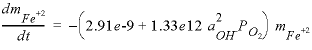, (22)

where t is time in seconds,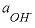is the activity of the hydroxyl ion,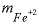is the total molality of ferrous iron in solution, and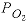is the oxygen partial pressure (atm).

The time for complete oxidation of ferrous iron is a matter of minutes in an aerated solution when pH is above 7.0. However, Fe +3 forms solute complexes with OH - and it may also precipitate as iron oxyhydroxides, so that pH decreases during oxidation. Because the rate has quadratic dependence on the activity of OH - , the oxidation rate rapidly diminishes as pH decreases. The rate equation is highly non-linear in an unbuffered solution and must be integrated numerically. This example models a reaction vessel with 10 mmol/kgw NaCl and 0.1 mmol/kgw FeCl 2 at pH = 7.0 through which air is bubbled; the change in solution composition over time is calculated.

The calculation requires the uncoupling of equilibrium among the Fe(2) and Fe(3) species. Two new “elements” are defined in SOLUTION_MASTER_SPECIES--“Fe_di”, which corresponds to Fe(2), and “Fe_tri”, which corresponds to Fe(3). The master species for these elements are defined to be Fe_di+2 and Fe_tri+3, and all solution species, phases, exchange species, and surface species must be rewritten using these new elements and master species. A few of the transcriptions are shown in table 28, which gives the partial input file for this example.

 `TITLE Example 9.--Kinetically controlled oxidation of ferrous ` ` iron. Decoupled valence states of iron.` `SOLUTION_MASTER_SPECIES` `Fe_di Fe_di+2 0.0 Fe_di 55.847` `Fe_tri Fe_tri+3 0.0 Fe_tri 55.847` `SOLUTION_SPECIES` `Fe_di+2 = Fe_di+2` ` log_k 0.0` `Fe_tri+3 = Fe_tri+3` ` log_k 0.0` `#` `# Fe+2 species` `#` `Fe_di+2 + H2O = Fe_diOH+ + H+` ` log_k -9.5` ` delta_h 13.20 kcal` `#` `#... and also other Fe+2 species` `#` `#` `# Fe+3 species` `#` `Fe_tri+3 + H2O = Fe_triOH+2 + H+` ` log_k -2.19` ` delta_h 10.4 kcal` `#` `#... and also other Fe+3 species` `#` `PHASES` `Goethite` ` Fe_triOOH + 3 H+ = Fe_tri+3 + 2 H2O` ` log_k -1.0` `END` `SOLUTION 1` ` pH 7.0` ` pe 10.0 O2(g) -0.67` ` Fe_di 0.1` ` Na 10.` ` Cl 10. charge` `EQUILIBRIUM_PHASES 1` ` O2(g) -0.67` `RATES` `Fe_di_ox` ` -start` ` 10 Fe_di = TOT("Fe_di")` ` 20 if (Fe_di <= 0) then goto 200` ` 30 p_o2 = SR("O2(g)")` ` 40 moles = (2.91e-9 + 1.33e12 * (ACT("OH-"))^2 * p_o2) * Fe_di * TIME` ` 200 SAVE moles` ` -end` `KINETICS 1` `Fe_di_ox` ` -formula Fe_di -1.0 Fe_tri 1.0` ` -steps 100 400 3100 10800 21600 5.04e4 8.64e4 1.728e5 1.728e5 1.728e5 1.728e5 ` ` -step_divide 1e-4` `INCREMENTAL_REACTIONS true` `SELECTED_OUTPUT` ` -file ex9.sel` ` -reset false` `USER_PUNCH` ` -headings Days Fe(2) Fe(3) pH si_goethite` ` 10 PUNCH SIM_TIME / 3600 / 24, TOT("Fe_di")*1e6, TOT("Fe_tri")*1e6, -LA("H+"),\` ` SI("Goethite")` `USER_GRAPH Example 9` ` -headings _time_ Fe(2) Fe(3) pH` ` -chart_title "Oxidation of Ferrous Iron"` ` -axis_titles "Time, in days" "Micromole per kilogram water" "pH"` ` -axis_scale secondary_y_axis 4.0 7.0 1.0 0.5` ` -start` ` 10 GRAPH_X TOTAL_TIME / 3600 / 24 ` ``` 20 GRAPH_Y TOT("Fe_di")*1e6, TOT("Fe_tri")*1e6 ``` ` 30 GRAPH_SY -LA("H+")` ` -end` `END`

The SOLUTION data block defines a sodium chloride solution that has 0.1 mmol/kgw ferrous iron (Fe_di) and is in equilibrium with atmospheric oxygen. The EQUILIBRIUM_PHASES data block specifies that all batch-reaction solutions will also be in equilibrium with atmospheric oxygen; thus, there is a continuous supply of oxygen for oxidation of ferrous iron.

In the RATES data block, the rate expression is designated with the name “Fe_di_ox” and defined according to equation 22. Note the use of the special Basic function “TOT” to obtain the total concentration (molality) of ferrous iron (line 10), “SR” to obtain the saturation ratio, or, in the case of a gas, the partial pressure, here of oxygen (line 30), and “ACT” to obtain the activity of OH - (line 40). Line 40 defines the moles of reaction. Notice also that the variable moles is calculated by multiplying the rate times the current time interval (TIME) and that the rate definition ends with a SAVE statement. The SAVE and TIME statements must be included in a rate definition; they specify the moles that reacted over the time (sub-)interval. The interval given by TIME is an internal PHREEQC variable that is adapted automatically by the code to obtain the required accuracy of the integration.

In the KINETICS data block, the rate expression named “Fe_di_ox” is invoked and parameters are defined. When the rate name in the KINETICS data block is identical to a mineral name that is defined under PHASES, the stoichiometry of that mineral will be used in the reaction. However, because no mineral is associated with the rate name of this example, the identifier -formula must be used to specify the reaction stoichiometry. The reaction involves loss of Fe_di [equivalent to Fe(2)] from solution as indicated by the stoichiometric coefficient of -1.0. The loss is balanced by a gain in solution of Fe_tri [equivalent to Fe(3)] with a stoichiometric coefficient of +1.0. Note that the formula contains only the elements for which the mass changes in the system. Thus, the overall kinetic reaction of the example is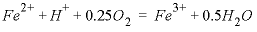, but the reaction of protons and oxygen to form water does not change the total mass of hydrogen or oxygen in the system. Hydrogen and oxygen are therefore not included in the formula. In table 28, the phase O2(g) in EQUILIBRIUM_PHASES allows dissolved oxygen to be maintained in equilibrium with atmospheric oxygen gas. In a system closed to oxygen, the dissolved oxygen would be partly consumed.

The identifier -steps in the KINETICS data block gives the time step(s) over which the kinetic reactions must be integrated. When INCREMENTAL_REACTIONS true is used, each time step increments the total time to be simulated, and the results from the previous time step are used as the starting point for the current time step.

The SELECTED_OUTPUT data block specifies the file name of the selected-output file and eliminates all default printing to that file ( -reset false). The only output to the selected-output file in this example is defined with the USER_PUNCH data block. The Basic program in USER_PUNCH specifies that the following be printed after each kinetic time step ( -steps defines 11 kinetic time steps): the cumulative time of the simulation, in days; the total ferrous and ferric iron, in μ mol/kgw; the pH; and the saturation index of goethite. The results also are plotted with USER_GRAPH, and the points can be saved to a file by right-clicking the mouse when the cursor is inside the chart area.

When the input file is run, two warning messages are generated during the integration. If the integration time interval is too large, it is possible that the initial estimates of kinetic reaction increments produce negative solution concentrations. When this happens, the program prints a warning message, decreases the size of the time interval, and restarts the integration. The messages are warnings, not errors, and the program successfully completes the calculation. It is possible to eliminate the warning messages by reducing the initial integration interval. No warning messages are printed if the identifier -step_divide 100 is used (KINETICS), which divides the initial (overall) time step by 100. Likewise, no warning messages are printed if the identifier -step_divide 1e-7 is used, which causes the reaction increment to be less than 1×10 -7 mol. The former approach, with -step_divide 100, is usually preferable because, although initial reaction increments are compelled to be small, later in the integration, large reaction increments are possible. Using -step_divide 1e-7 forces reaction increments to remain small throughout the entire integration, and in this example, the run time is about 5 times longer than using -step_divide 100, and about 10 times longer than not using -step_divide at all. Figure 10 shows the concentration of total Fe(2), total Fe(3), and pH in the reaction vessel over the 10 days of the simulation. It can be seen that the pH rapidly decreases at the beginning of the reaction. The slope of Fe(2) against time is initially steep, but lessens as the reaction progresses, which is consistent with equation 22.When the experiment is performed in reality in an unbuffered solution, it is noted that the pH initially rises. This rise in pH is consistent with slowly forming hydroxy-complexes of Fe(3). Because the oxidation reaction by itself consumes protons, the pH would initially rise if the hydroxy-complexes that lower the pH form slowly. Such kinetic formation of aqueous complexes also could be included in PHREEQC simulations, but it would require that the hydroxy-complexes of Fe(3) also be defined by using a separate SOLUTION_MASTER_SPECIES and that a rate expression be defined for the kinetic formation of the complexes.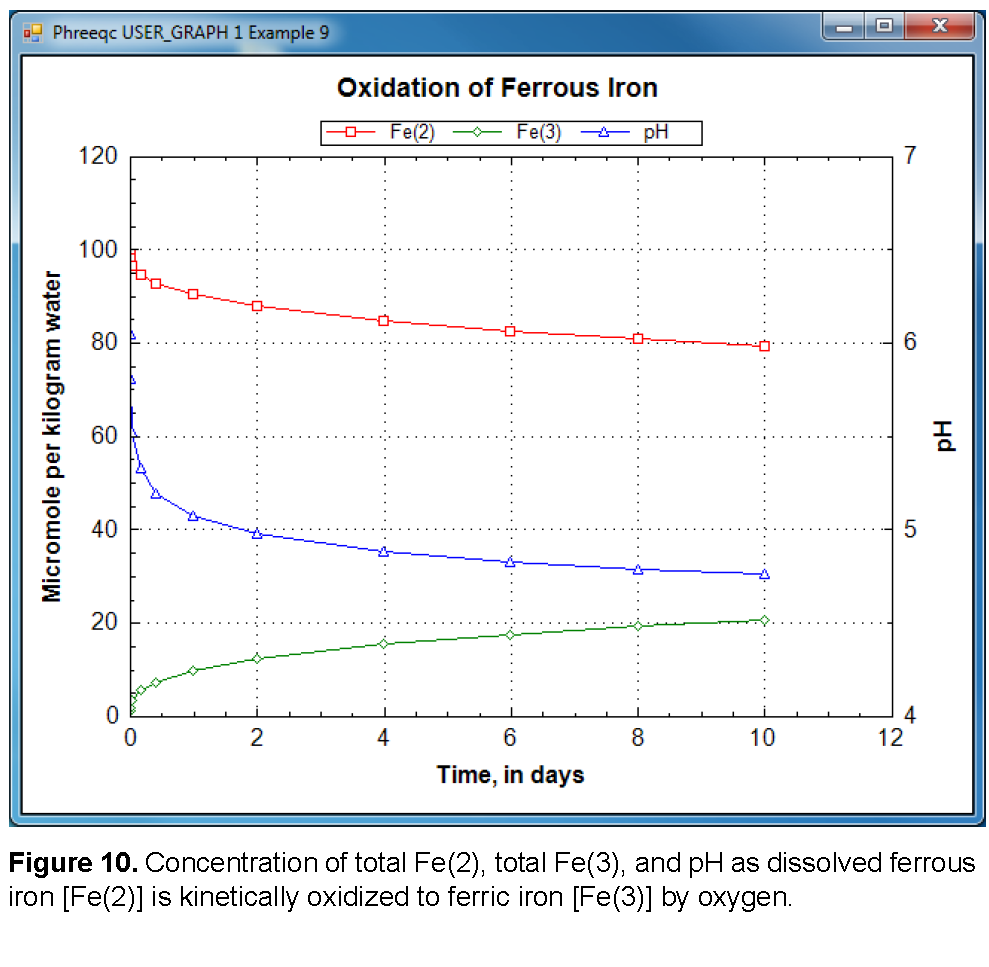| Next || Previous || Top |